edHelper subscribers - Create a new printable

Number of Keys
 Select the number of different printables: 1 key 2 keys 3 keys 4 keys 5 keys

Number of Pages (for each key)
 Select the number of pages: 1 page 2 pages 3 pages 4 pages

Include an answer key (answer keys will be at the end of the printable)
High School Geometry
High School Geometry
Math

 Name _____________________________Date ___________________ (Key 1 - Answer ID # 0932244)
1.

State whether the polygon is best described as equiangular, equilateral, regular, or none of these.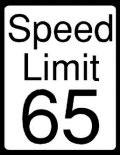2.

State whether the figure is a polygon. If it is, identify the polygon and state whether it is convex or concave. If it is not, explain why.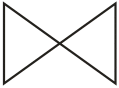3.

State whether the figure is a polygon. If it is, identify the polygon and state whether it is convex or concave. If it is not, explain why.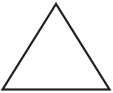4.

State whether the figure is a polygon. If it is, identify the polygon and state whether it is convex or concave. If it is not, explain why.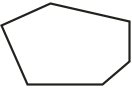5.

State whether the polygon is best described as equiangular, equilateral, regular, or none of these.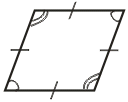6.

State whether the figure is a polygon. If it is, identify the polygon and state whether it is convex or concave. If it is not, explain why.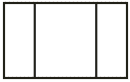edHelper subscribers - Create a new printable

Number of Keys
 Select the number of different printables: 1 key 2 keys 3 keys 4 keys 5 keys

Number of Pages (for each key)
 Select the number of pages: 1 page 2 pages 3 pages 4 pages